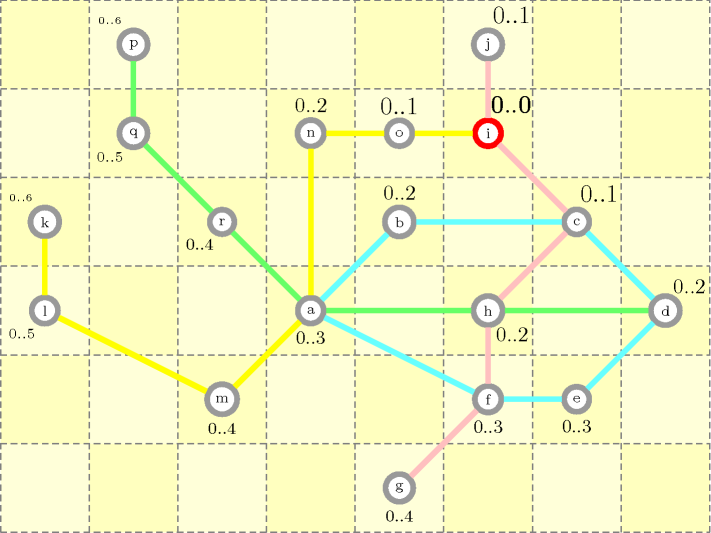### 3.7.151. Metro

modelling: metro A constraint that can be used for modelling the metro problem, i.e., finding the shortest distance from a given metro station to all other stations of the network.

Given an undirected graph $G=\left(V,E\right)$, with a non-negative distance attached to each edge of $E$, a conjunction of $\mathrm{𝚕𝚎𝚚}_\mathrm{𝚌𝚜𝚝}$ constraints was used by H. Simonis in order to illustrate how propagation for such a conjunction simulates a naïve version of Dijsktra algorithm for computing the shortest distance from a given vertex ${v}_{s}$ of $V$ to all other vertices. The potential source of inefficiency comes from the fact that, depending on the scheduling policy of the underlying constraint engine, an inequality constraint can be reconsidered several times before reaching the fixed point. The problem was modelled in the following way:

• To each vertex ${v}_{i}\in V$ we associate a distance variable ${D}_{i}$, which represents the domain range of the distance between vertex ${v}_{i}$ and vertex ${v}_{s}$.

• To each edge $\left({v}_{i},{v}_{j}\right)\in E$ we impose two inequality constraints ${D}_{i}\le {D}_{j}+{d}_{i,j}$ and ${D}_{j}\le {D}_{i}+{d}_{i,j}$, where ${d}_{i,j}$ corresponds to the distance attached to edge $\left({v}_{i},{v}_{j}\right)$. This restricts the maximum difference between the distances variables associated with the two extremities of edge $\left({v}_{i},{v}_{j}\right)$.

• Finally, we set the distance variable attached to vertex ${v}_{s}$ to 0. Propagating the inequalities constraints by using arc-consistency enforces the maximum value of each distance variable ${D}_{i}$ to be equal to the shortest distance from vertex ${v}_{i}$ to ${v}_{s}$ when the fixed point is reached.

##### Figure 3.7.41. A metro map composed of four lines (a blue, a pink, a green and a yellow line) and the corresponding minimum and maximum values of the distance variables attached to each station, under the assumptions (1) that the distance attached to each connection is equal to 1 and (2) that we compute the shortest path from station i (in red); the font size used for displaying the bounds of a distance variable is inversely proportional to the length of the shortest path to station i.Figure 3.7.41 illustrates this problem on a metro map composed of four lines and 18 stations respectively labelled by a, b, $\cdots$, r. Its assumes that the distance associated with each connection is equal to 1. The figure displays the status (i.e., the minimum and maximum values) of the distance variables under the assumption that we want to compute the shortest path from station i. The inequalities constraints between the distance variables ${D}_{a},{D}_{b},\cdots ,{D}_{r}$ corresponding to this metro map are:

• (constraints attached to the connections of the blue metro line)

•   ${D}_{a}\le {D}_{b}+1$,

•   ${D}_{b}\le {D}_{c}+1$,

•   ${D}_{c}\le {D}_{d}+1$,

•   ${D}_{d}\le {D}_{e}+1$,

•   ${D}_{e}\le {D}_{f}+1$,

•   ${D}_{f}\le {D}_{a}+1$,

•   ${D}_{b}\le {D}_{a}+1$,

•   ${D}_{c}\le {D}_{b}+1$,

•   ${D}_{d}\le {D}_{c}+1$,

•   ${D}_{e}\le {D}_{d}+1$,

•   ${D}_{f}\le {D}_{e}+1$,

•   ${D}_{a}\le {D}_{f}+1$.

• (constraints attached to the connections of the pink metro line)

•   ${D}_{g}\le {D}_{f}+1$,

•   ${D}_{f}\le {D}_{h}+1$,

•   ${D}_{h}\le {D}_{c}+1$,

•   ${D}_{c}\le {D}_{i}+1$,

•   ${D}_{i}\le {D}_{j}+1$,

•   ${D}_{f}\le {D}_{g}+1$,

•   ${D}_{h}\le {D}_{f}+1$,

•   ${D}_{c}\le {D}_{h}+1$,

•   ${D}_{i}\le {D}_{c}+1$,

•   ${D}_{j}\le {D}_{i}+1$.

• (constraints attached to the connections of the green metro line)

•   ${D}_{p}\le {D}_{q}+1$,

•   ${D}_{q}\le {D}_{r}+1$,

•   ${D}_{r}\le {D}_{a}+1$,

•   ${D}_{a}\le {D}_{h}+1$,

•   ${D}_{h}\le {D}_{d}+1$,

•   ${D}_{q}\le {D}_{p}+1$,

•   ${D}_{r}\le {D}_{q}+1$,

•   ${D}_{a}\le {D}_{r}+1$,

•   ${D}_{h}\le {D}_{a}+1$,

•   ${D}_{d}\le {D}_{h}+1$.

• (constraints attached to the connections of the yellow metro line)

•   ${D}_{k}\le {D}_{l}+1$,

•   ${D}_{l}\le {D}_{m}+1$,

•   ${D}_{m}\le {D}_{a}+1$,

•   ${D}_{a}\le {D}_{n}+1$,

•   ${D}_{n}\le {D}_{o}+1$,

•   ${D}_{o}\le {D}_{i}+1$,

•   ${D}_{l}\le {D}_{k}+1$,

•   ${D}_{m}\le {D}_{l}+1$,

•   ${D}_{a}\le {D}_{m}+1$,

•   ${D}_{n}\le {D}_{a}+1$,

•   ${D}_{o}\le {D}_{n}+1$,

•   ${D}_{i}\le {D}_{o}+1$.